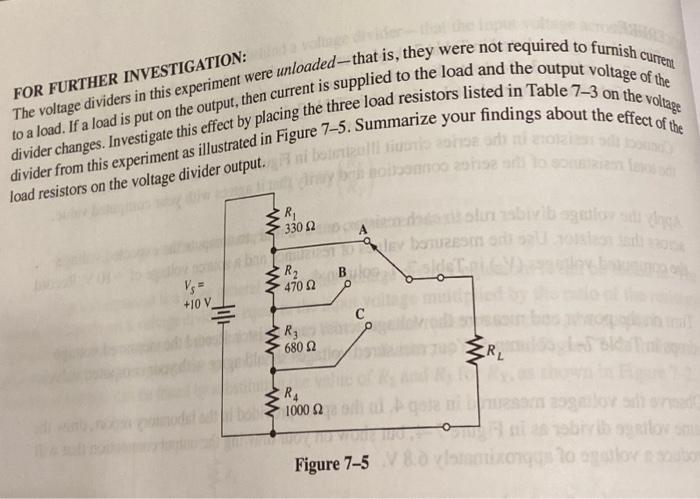# Question Curren FOR FURTHER INVESTIGATION: to a load. If a load is put on the output, then current is supplied to the load and the output voltage of the The voltage dividers in this experiment were unloaded--that is, they were not required to furnish divider changes. Investigate this effect by placing the three load resistors listed in Table 7-3 on the voltage divider from this experiment as illustrated in Figure 7-5. Summarize your findings about the effect of the ml bottigt Bobomo load resistors on the voltage divider output. R 330 Ω olub begalo a bomo } R2 4702 +10 V Hili с R; 6802 W W CRL RA 1000 Ω Figure 7-5 V Section to allow toVCFKSH The Asker · Electrical EngineeringTranscribed Image Text: Curren FOR FURTHER INVESTIGATION: to a load. If a load is put on the output, then current is supplied to the load and the output voltage of the The voltage dividers in this experiment were unloaded--that is, they were not required to furnish divider changes. Investigate this effect by placing the three load resistors listed in Table 7-3 on the voltage divider from this experiment as illustrated in Figure 7-5. Summarize your findings about the effect of the ml bottigt Bobomo load resistors on the voltage divider output. R 330 Ω olub begalo a bomo } R2 4702 +10 V Hili с R; 6802 W W CRL RA 1000 Ω Figure 7-5 V Section to allow to
More
Transcribed Image Text: Curren FOR FURTHER INVESTIGATION: to a load. If a load is put on the output, then current is supplied to the load and the output voltage of the The voltage dividers in this experiment were unloaded--that is, they were not required to furnish divider changes. Investigate this effect by placing the three load resistors listed in Table 7-3 on the voltage divider from this experiment as illustrated in Figure 7-5. Summarize your findings about the effect of the ml bottigt Bobomo load resistors on the voltage divider output. R 330 Ω olub begalo a bomo } R2 4702 +10 V Hili с R; 6802 W W CRL RA 1000 Ω Figure 7-5 V Section to allow to